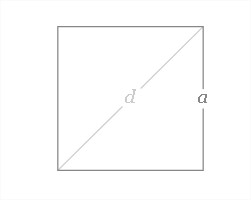# C Program To Calculate Perimeter Of Square | C Programs

C Program to Calculate the perimeter of a square – In this distinct article, we will brief in on the multiple ways to calculate the perimeter of a square in C Programming.

In this blog, we have given suitable examples and sample programs for you. The compiler has also been added in order to understand the codes little better.

The ways in which the calculations can be done are as follows:

• Using Standard Method
• Using Functions
• Using Pointers
• Using Macros

As we all know, a square is a 2-dimensional quadrilateral figure whose sides are all equal and the opposite sides are parallel. Each angle of a square is equal to 90 degrees.

This is what a square looks like:As you can see, this square has “a” as its side length. Hence, the perimeter of a square is as follows:

Perimeter = a + a + a + a = 4a.

Thus, the methods with which the perimeter of a square is calculated in C programming are as follows:

## Using Standard Method

1)The formula for the perimeter of a square is perimeter=4*s.

2)We are calculating the perimeter using the same formula by substituting the value “side” which is input to this program

3)The calculated value stored into the variable “perimeter”

output:

### Using Functions

1)Here the function is float perimeter(float s), which is having float variable as argument and it returns the float value

2)We are calling the function using perimeter(s), here “s” is float type variable, this “s” will pass to the perimeter

output:

#### Using Pointers

1)Here we are passing the addresses of s,p variables using function perimeter(&s,&p).

2)The values at that address &s,&p will store into pointer variables *s,*p

3)The function void perimeter(float *s, float *p), calculate the perimeter of the square and that value will store into pointer variable *p

output:

##### Using Macros

1)the formula 4*s was defined with the name area(s)

2)We have code as p=area(s),but area(s) means 4*s.

3)So p=4*s will be calculated and store into p

output:
x

## C Program To Print Number Of Days In A Month | Java Tutoring

C program to input the month number and print the number of days in that ...